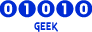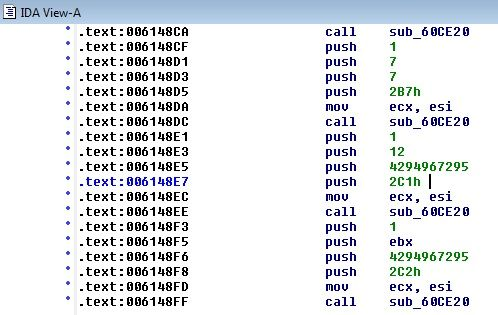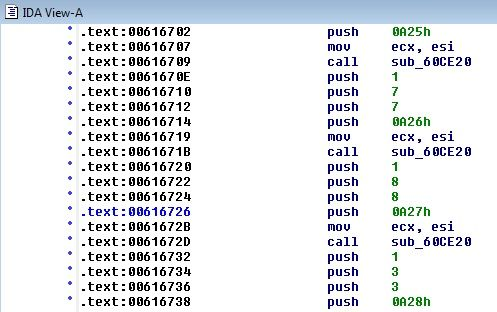## Usage: Packet Extraction Evaluation Kit (PEEK)

Do you have a question or problem? Read this forum first! Someone has probably already asked the same thing in the past. Do not ASK questions here!

Moderators: Moderators, Documentation Writers

Message
Author
jokinvipo
HumanPosts: 20
Joined: 20 Mar 2011, 08:15
Noob?: Yes

### Re: Usage: Packet Extraction Evaluation Kit (PEEK)

@4epT

I' used the Packet Extractor-1.1.0 and RO Tools to extract recvpackets and the PEEK but is not working.

Can you give me that big help extracting the rcvpackets?
I found the server info and im logging in but i cant move or do anything.

The server is http://remember-ro.net/site/

Thanks alot4epT
DevelopersPosts: 592
Joined: 30 Apr 2008, 14:17
Noob?: No
Location: Moskow (Russia)
Contact:

### Re: Usage: Packet Extraction Evaluation Kit (PEEK)

jokinvipo, from what file you are trying to get the package?
All my posts are made by machine translator!
¤ Manual ¤ Anti BotKiller ¤ Packet Extractor v3 ¤jokinvipo
HumanPosts: 20
Joined: 20 Mar 2011, 08:15
Noob?: Yes

### Re: Usage: Packet Extraction Evaluation Kit (PEEK)

I tried to extract the launchers but is not working.

i only need the rcvpackets for this kRO_Sakexe_2008_09_10a then my bot should run w/o problems.

Can you give me a hand?

Thanks

4epT
DevelopersPosts: 592
Joined: 30 Apr 2008, 14:17
Noob?: No
Location: Moskow (Russia)
Contact:

### Re: Usage: Packet Extraction Evaluation Kit (PEEK)

jokinvipo, use "remember-ro.bin" and "openkore-packet-extractor-1.1.0"
All my posts are made by machine translator!
¤ Manual ¤ Anti BotKiller ¤ Packet Extractor v3 ¤Shakti-lar
NoobPosts: 8
Joined: 17 Jan 2015, 20:45
Noob?: Yes

### Re: Usage: Packet Extraction Evaluation Kit (PEEK)

sir, i got Unknown switch
# Packet Parser: Unknown switch: 02C1
# Packet Tokenizer: Unknown switch: 0A27

a tried already with tools from http://wiki.openkore.com/index.php/Packet_Length_Extractor, but failed (over 6MB)
also tried with PEEK, could you help...

then i tried with IDA, and search text manually02C1 4294967295 12 1
0A27 8 8 1

but the value seem to high
https://www.mediafire.com/?p11m940g4261z1g

tmrcik
NoobPosts: 17
Joined: 06 Feb 2009, 07:28
Noob?: Yes

### Re: Usage: Packet Extraction Evaluation Kit (PEEK)

Hello guys, I'm trying to use this application. But it seems I'm kinda confused with the exes that which I am going to use. I've read times over times but I'm not getting this. So I'll write down what I have and I would like to know what I have to do. (I'm trying to log on to fRo servers btw via OpenKore)

I've extracted PEEK into the RO folder itself with all the files that are included within PEEKHere are the original game files that I am choosing for PEEK to work on.After this point when I hit "Start Analyzer" button, I get the error message;I'm sure that I'm doing something wrong, but I cannot figure it out yet. If you guys can help me kindly, I'll be glad ! (Also will post the fRO's updated recvtables if I succeed. Or more than that I can also share the files if needed.)

chiky
HumanPosts: 22
Joined: 22 Feb 2013, 10:18
Noob?: Yes

### Re: Usage: Packet Extraction Evaluation Kit (PEEK)

i've tried to extract this but got this packet error.here's the exe.
http://www.4shared.com/file/9xnj0iZ9ce/LeafRO.html

4epT
DevelopersPosts: 592
Joined: 30 Apr 2008, 14:17
Noob?: No
Location: Moskow (Russia)
Contact:

### Re: Usage: Packet Extraction Evaluation Kit (PEEK)

Shakti-lar, "Unknown switch" - This means that you are using is not the right serveType. Check your file recvpackets.txt
you have to find "02C1 0" and "0A27 8". By the way how you extracted the packages?

tmrcik, Try to use other extractors.

All my posts are made by machine translator!
¤ Manual ¤ Anti BotKiller ¤ Packet Extractor v3 ¤andrecastro
NoobPosts: 6
Joined: 26 Jan 2015, 12:58
Noob?: Yes

### Re: Usage: Packet Extraction Evaluation Kit (PEEK)

Hello people of this community =D

https://github.com/ThemonChan/PEEK
http://nbsxey3vnrsxgltxom.cmle.ru/board ... -analyzer/

Mini guide:
2. run PEEK.exe
3. click "Browse" and select your client file (not necessarily exe!)
4. click "Start Analyzer"
5. close PEEK
6. open folder: ..\PEEK\Output\
7. run 1.bat
8. file will appear: recvpackets.txt

i followed this guide to get the recvpackets.txt but when i get to step 7, i cant find any bat file in the entire PEEK folder.
Maybe its something you have to download from the second link, but i cant access that site. It makes me go to this site: http://cameleo.ru/?msg=edsina wich is in some language i cant understand and the google translations doesnt make any sense... =\
Including the bat file that is required in step 7?

Anyway i still get a recvpackets_20120515.txt file wich contains the following:

Code: Select all

``//Extracted With DiffGen2[Old_Table]0x0187 = 60x0081 = 30x01c6 = 40x01c7 = 20x0064 = 550x0069 = -10x006a = 230x01db = 20x01dc = -10x01dd = 470x01fa = 480x0204 = 180x01f1 = -10x0200 = 260x01be = 20x01bf = 30x0065 = 170x0066 = 30x0067 = 370x0970 = 310x020d = -10x006b = -10x006c = 30x006d = 1460x006e = 30x0071 = 280x0068 = 460x01fb = 560x006f = 20x0070 = 30x02ca = 30x009b = 340x0082 = 20x00a7 = 90x0190 = 230x00f3 = -10x0089 = 110x007d = 20x0090 = 70x0099 = -10x019c = -10x0085 = 100x00cc = 60x00ce = 20x00f5 = 110x0116 = 170x009f = 200x00a9 = 60x00ab = 40x00b8 = 70x00b9 = 60x00b2 = 30x00ba = 20x00bb = 50x00bf = 30x00c1 = 20x00c5 = 70x00c8 = -10x00c9 = -10x0096 = -10x00cf = 270x00d0 = 30x00d3 = 20x00d5 = -10x00d9 = 140x00de = -10x00e0 = 300x00e2 = 260x00e3 = 20x00e4 = 60x00e6 = 30x00e8 = 80x00eb = 20x00ed = 20x00ef = 20x0094 = 190x00f7 = 170x0193 = 20x00f9 = 260x01e8 = 280x00fc = 60x00ff = 100x0102 = 60x0100 = 20x0103 = 300x0108 = -10x0112 = 40x0072 = 220x0113 = 250x0118 = 20x011b = 200x011d = 20x0126 = 80x0127 = 80x0128 = 80x0129 = 80x012a = 20x012e = 20x012f = -10x01b2 = -10x0134 = -10x0130 = 60x0138 = 30x013f = 260x0140 = 220x0143 = 100x0146 = 60x0178 = 40x017a = 40x017c = 60x01fd = 150x018a = 40x018e = 100x007e = 1050x0197 = 40x0198 = 80x01ae = 40x025b = 60x01af = 40x01b9 = 60x01cd = 300x01ce = 60x0443 = 80x01cf = 280x01d0 = 80x01e1 = 80x01d1 = 140x01d2 = 100x008c = 140x0095 = 300x0195 = 1020x00a2 = 140x0175 = 60x0176 = 1060x0079 = 530x019d = 60x014c = -10x014d = 20x014e = 60x014f = 60x0150 = 1100x01b6 = 1140x0151 = 60x0152 = -10x0153 = -10x0154 = -10x0166 = -10x0155 = -10x0156 = -10x0157 = 60x0159 = 540x015a = 660x015b = 540x015c = 900x015d = 420x015e = 60x015f = 420x0160 = -10x0161 = -10x0162 = -10x0163 = -10x0164 = -10x0165 = 300x0167 = 30x0168 = 140x0169 = 30x016a = 300x016b = 100x0149 = 90x014a = 60x014b = 270x016e = 1860x016f = 1820x017e = -10x017f = -10x0165 = 300x0166 = -10x0167 = 30x0168 = 140x0169 = 30x016a = 300x016b = 100x016c = 430x016c = 430x016d = 140x01f2 = 200x0170 = 140x0171 = 300x0172 = 100x0173 = 30x0174 = -10x0180 = 60x0181 = 30x0182 = 1060x0183 = 100x0184 = 100x0185 = 340x019e = 20x019f = 60x01a0 = 30x01a1 = 30x01a2 = 370x01a3 = 50x01a4 = 110x01a5 = 260x01a6 = -10x01a7 = 40x01a8 = 40x01a9 = 60x01aa = 100x01ca = 30x01b0 = 110x01b1 = 70x01ba = 260x01bb = 260x01bc = 260x01bd = 260x01c0 = 20x01c1 = 140x01c2 = 100x01d3 = 350x01d5 = -10x01d4 = 60x01df = 60x01f3 = 100x0284 = 140x01ff = 100x01ed = 20x01e7 = 20x01b7 = 60x01f7 = 140x01e3 = 140x01cb = 90x01f9 = 60x01e5 = 60x0201 = -10x0369 = 260x0203 = 100x0205 = 260x0206 = 110x0207 = 340x0208 = 140x0209 = 360x020a = 100x020e = 320x020f = 100x0210 = 220x0212 = 260x0213 = 260x0214 = 420x0215 = 60x0216 = 60x0217 = 20x0218 = 20x0225 = 20x0966 = 40x0219 = 2820x021a = 2820x0226 = 2820x0282 = 2840x021b = 100x021c = 100x0224 = 100x0280 = 120x0285 = 60x0286 = 40x021d = 60x021e = 60x021f = 660x0222 = 60x0221 = -10x0220 = 100x0223 = 80x0073 = 110x0074 = 30x0075 = -10x0076 = 90x0077 = 50x0078 = 550x007a = 580x007b = 600x007c = 440x007f = 60x0080 = 70x0083 = 20x0084 = 20x0086 = 160x0087 = 120x0088 = 100x08cd = 100x008a = 290x008b = 230x008d = -10x008e = -10x0091 = 220x0092 = 280x0093 = 20x0097 = -10x0098 = 30x009a = -10x009c = 90x009d = 170x009e = 170x00a0 = 230x00a1 = 60x00a3 = -10x00a4 = -10x00a5 = -10x00a6 = -10x00a8 = 70x00aa = 90x00ac = 70x00ae = -10x00af = 60x00b0 = 80x00b1 = 80x00b3 = 30x00b4 = -10x00b5 = 60x00b6 = 60x00b7 = -10x00bc = 60x00bd = 440x00be = 50x00c0 = 70x00c2 = 60x00c3 = 80x00c4 = 60x00c6 = -10x00c7 = -10x00ca = 30x00cb = 30x00cd = 30x00d1 = 40x00d2 = 40x00d4 = -10x00d6 = 30x00d7 = -10x00d8 = 60x00da = 30x00db = -10x00dc = 280x00dd = 290x00df = -10x00e1 = 300x00e5 = 260x00e7 = 30x00e9 = 190x00ea = 50x00ec = 30x00ee = 20x00f0 = 30x00f1 = 20x00f2 = 60x00f4 = 210x00f6 = 80x00f8 = 20x00fa = 30x00fb = -10x00fd = 270x00fe = 30[Encryption]Key1 = 0x4a715ef9Key2 = 0x79103e4fKey3 = 0x405c1238``

I cutted most part of the Old Table from the code in order to make it easy to read the post.

This didnt work as a recvpackets.txt for my server so i looked up and in the 2nd post of this topic the Moderator Skylord said there was a need to place a filename.pl file with the code he shared on the output paste of PEEK. and then run "it" in order to make the convertion automatic.

Save as filename.pl '-' Inside Output folder of PEEK. Now run it!

Should i place this .pl file in the Output paste before i run the PEEK?
If yes, should i create the paste Output to put it there or...?
If no, what is the "it" that i should run?

irzam69
NoobPosts: 1
Joined: 30 Jan 2015, 20:54
Noob?: No

hi....